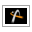AstroGrav Help /Menus /Tools /Calculate Orbits...Version 5.0.1

# Tools / Calculate Orbits...   ()

Operating Systems: All
Window Types: All
Simulation Types: All

### Summary

Provides a choice of two different ways of calculating orbital elements in the frontmost simulation.

### Description

When you select this command, a small dialog will be displayed allowing you to choose from the following two different ways of calculating orbital elements in the frontmost simulation.

• Calculate orbital elements relative to the parent object only.

• Calculate orbital elements relative to the barycenter of all inferior objects.

To use the solar system as an example, if orbital elements are calculated relative to the parent object only, then all planets will have their orbital elements calculated relative to the Sun. If orbital elements are calculated relative to the barycenter of all inferior objects, then Mercury will have its orbital elements calculated relative to the Sun (as before), Venus will have its orbital elements calculated relative to the barycenter of the Sun and Mercury, Earth will have its orbital elements calculated relative to the barycenter of the Sun and Mercury and Venus, and so on.

A situation in which it would be best to calculate orbital elements relative to the barycenter of all inferior objects is if you were interested in studying the long-term evolution of the planets' orbital elements, say by evolving with a 1,000 year time step. If you calculate orbital elements relative to the Sun, then the long-term changes in orbital elements are swamped by the much larger oscillations caused by the Sun's movement relative to the solar system barycenter - particularly an 11.86 year oscillation associated with Jupiter. Calculating orbital elements relative to the barycenter of all inferior objects removes these unwanted oscillations entirely.

If you use the Edit / Import Objects... command to import comets or asteroids into a solar system simulation, the orbital elements of imported objects will exactly match the orbital elements of the import tables if and only if orbital elements are calculated relative to the Sun. This is because the orbital elements of import tables come directly from websites that always calculate orbital elements relative to the Sun, and if you want orbital elements calculated relative to the barycenter of all inferior objects, then AstroGrav must make the appropriate conversion.

Calculating orbital elements relative to the parent only works poorly in situations in which there is not a dominant object. For this reason, it is best to calculate orbital elements relative to the barycenter of all inferior objects when you're interested in a family in which the mass is not concentrated in the central object. To again use the solar system as an example, if orbital elements are calculated relative to the parent object only, then Pluto's moons (except for Charon) will appear to have misleading and widely varying orbital elements, because Charon is of significant mass compared with Pluto. Switching to calculating orbital elements relative to the barycenter of all inferior objects solves this problem. To give another example, any simulation that contains only objects with similar masses (such as a globular cluster) should have orbital elements calculated relative to the barycenter of all inferior objects.

• Edit / Undo()that undoes the latest change to the frontmost simulation.

• Edit / Redo(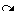)that redoes the most recently undone change to the frontmost simulation.

• Edit / Edit Object...(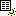)that edits the currently selected object in the active window.

• Edit / Add Object...(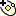)that adds a new object to the frontmost simulation.

• Edit / Add Family...(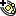)that adds a new family of objects to the frontmost simulation.

• Edit / Import Objects...(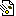)that imports comets and asteroids from the websites of NASA's Jet Propulsion Laboratory and the IAU's Minor Planet Center.